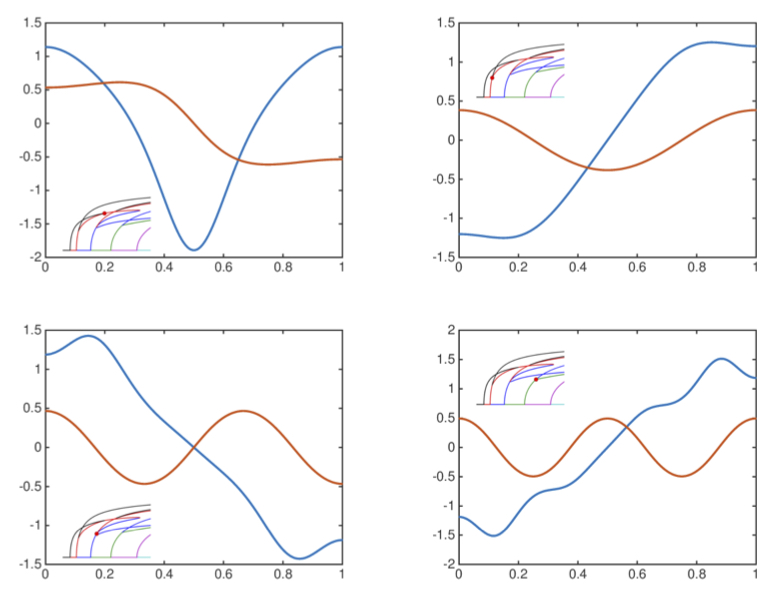### Rigorous continuation of bifurcation points in the diblock copolymer equation

Jean-Philippe Lessard, Evelyn Sander and Thomas Wanner.

We consider the problem of rigorously computing continuous branches of
bifurcation points of equilibria in the one-dimensional diblock copolymer
model. We apply the method both to fold points and to pitchfork bifurcations
which are forced through symmetries in the equation.

The paper in its pdf form can be found here.

Here are the MATLAB codes to perform the computer-assisted proofs of Theorem 6 and Theorem 7.
The codes require installing and starting the interval arithmetic package INTLAB.